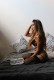# 7 Segment Decoder Implementation, Truth Table, Logisim Diagram

7 Segment Decoder Implementation, Truth Table, Logisim Diagram:

### 7 Segment Decoder:

For reference check this Wikipedia link.

### Pictures:

(Wikipedia CC BY-SA 2.5)

### Explanation:

Before we start implementing we first need to check if it is common anode or common cathode. If it is common anode then 3rd pin in both top and bottom are VCC. But if it is we should connect 3rd pin in both top and bottom to ground.

Pins:

show top pins then bottom pins ( Dot side is down ).

```        Pin1   Pin2   Pin3   Pin4   Pin5
Top:     g      f    vcc/GND   a     b
Bottom:  e      d    vcc/GND   c     dp
```

### Truth Table:

From here we can get minimized expressions for a, b, c, d, e, f, g using K-MAP. Here we only need value 0 though 9 rest are don’t care terms. Using those don’t care terms we will try to maximum ones first.

### K-Map (Karnaugh map) for ‘a’:

We can follow similar procedure for the rest. K map for ‘a’ can be created by taking the ‘a’ column from the table above and setting the value 0 / 1 to corresponding location in the table. For example to display 0 ( 0000 )  ‘a’ is always 1. Similarly to display 1 ‘a’ is always 0. For value ( 10 – 16 ) we don’t care about them so they are used as don’t care term in k map.

### Using Logisim to generate circuit diagram:

• ##### Go to Window -> Computational AnalysisNow input D, C, B, A as in which case D is MSB and A is LSB. Otherwise A, B, C, D can also be inputs and MSB, LSB will be opposite.
• ##### Now input D, C, B, A as in which case D is MSB and A is LSB. Otherwise A, B, C, D can also be inputs and MSB, LSB will be opposite.Now input the table given above in the table tab. We can just input values by single or double clicks.
• ##### Now input the table given above in the table tab. We can just input values by single or double clicks.k map for each of the variables / outputs can be seen herename the circuit and tick use two input gates, if you use two input gates otherwise its not needed.

## 3 thoughts on “7 Segment Decoder Implementation, Truth Table, Logisim Diagram”

1.Bryan Joshua says:

Good Day Sir. Can’t you help me for decoder sir, and how to k map?

Like

1.quickgrid says:

A 7 segment decoder can show maximum 9 in decimal format ( It can also show A to F in case of hexadecimal ). Using 3 bits the maximum number we can represent is 7. So in order to show 8, 9 on display you need 4 bits.

Just make K Map for all the inputs of the 7 segment decoder using the table. I have only shown the ‘a’ column. Now do it for all other columns. Using 4 bits$2^4$ combinations possible which is 16 from 0 to 15. Since a single 7 segment decoder can show only a single digit and my display format is decimal so use don’t care for 10 to 15. I haven’t showed the don’t care terms.

In case you don’t know how k map filled, You can easily find the k map for each input by inputting the table in logisim as I have shown.

Like

2.ZhengCaiZhen says:

very useful. thanks

Like